## Example Questions

### Example Question #33 : Triangles

One side of an equilateral triangle is equal toQuantity A: The area of the triangle.

Quantity B:Possible Answers:

The two quantities are equal.

The relationship cannot be determined.

Quantity B is greater.

Quantity A is greater.

Correct answer:

Quantity B is greater.

Explanation:

To find the area of an equilateral triangle, notice that it can be divided into two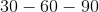triangles: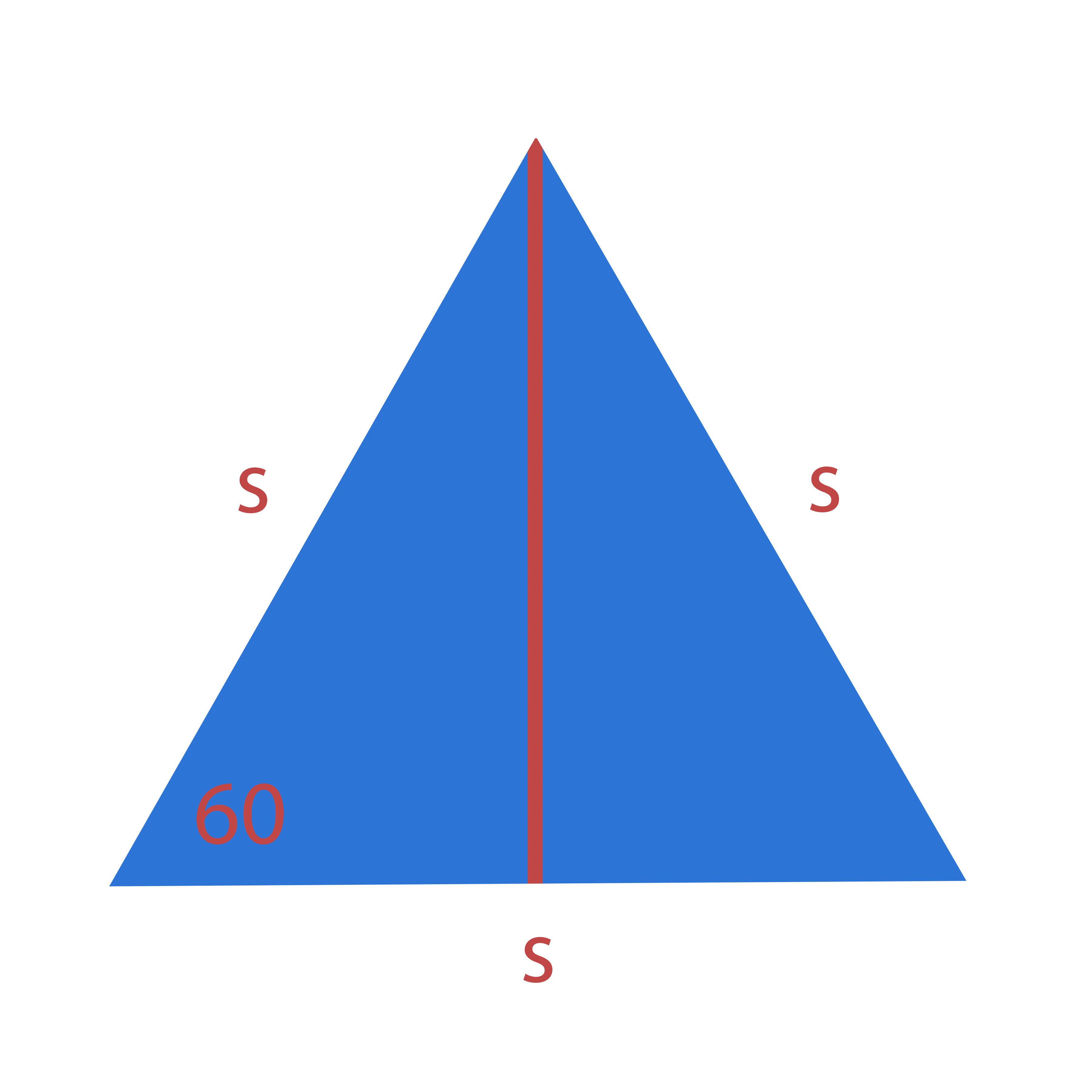The ratio of sides in atriangle is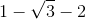, and since the triangle is bisected such that thedegree side is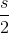, thedegree side, the height of the triangle, must have a length of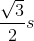.

The formula for the area of the triangle is given as: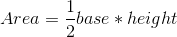So the area of an equilateral triangle can be written in term of the lengths of its sides as: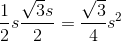For this particular triangle, since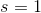, its area is equal to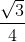.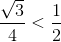If the relation between ratios is hard to visualize, realize that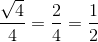### Example Question #34 : Triangles

If the area of an equilateral triangle is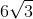, what is the height of the triangle?

Possible Answers: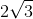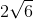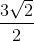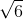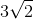Correct answer:Explanation:

The area of an equilateral triangle is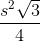.

So let's set-up an equation to solve for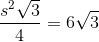Cross multiply.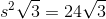The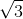cancels out and we get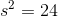.

Then take square root on both sides and we get. To find height, we need to realize by drawing a height we createtriangles.

The height is opposite the angle. We can set-up a proportion. Side oppositeisand the side of equilateral triangle which is oppositeis.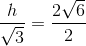Cross multiply.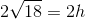Divide both sides by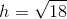We can simplify this by factoring out a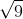to get a final answer of### Example Question #41 : Triangles

Quantity A: The height of an equilateral triangle with an area of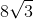Quantity B:Which of the following is true?

Possible Answers:

The two quantities are equal.

Quantity A is greater.

The relationship cannot be determined.

Quantity B is greater.

Correct answer:

Quantity B is greater.

Explanation:

This problem requires a bit of creative thinking (unless you have memorized the fact that an equilateral triangle always has an area equal to its side length times.

Consider the equilateral triangle: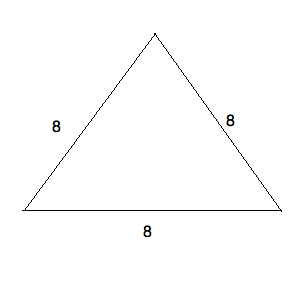Since this kind of triangle is a species of isoceles triangle, we know that we can drop down a height from the top vertex. This will create two equivalent triangles, one of which will look like: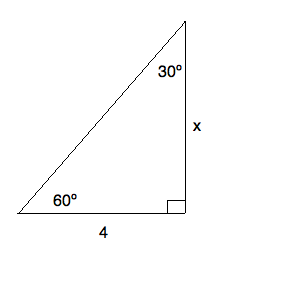This gives us a 30-60-90 triangle. We know that for such a triangle, the ratio of the side across from the 30-degree angle to the side across from the 60-degree angle is: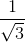We can also say, given our figure, that the following equivalence must hold: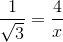Solving for, we get: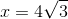Now, since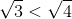, we know thatmust be smaller than. This means that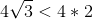or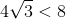. Quantity B is larger than quantity A.

### Example Question #42 : Triangles

Quantity A: The height of an equilateral triangle with perimeter of.

Quantity B:Which of the following is true?

Possible Answers:

The two quantities are equal.

Quantity A is larger.

The relationship cannot be determined.

Quantity B is larger.

Correct answer:

Quantity B is larger.

Explanation:

If the perimeter of our equilateral triangle is, each of its sides must be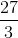or. This gives us the following figure: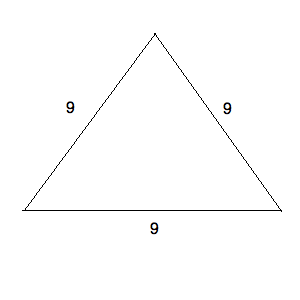Since this kind of triangle is a species of isoceles triangle, we know that we can drop down a height from the top vertex. This will create two equivalent triangles, one of which will look like: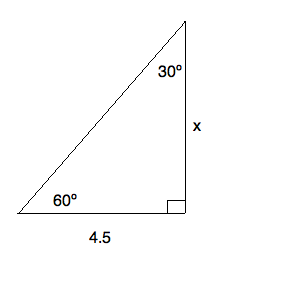This gives us a 30-60-90 triangle. We know that for such a triangle, the ratio of the side across from the 30-degree angle to the side across from the 60-degree angle is:Therefore, we can also say, given our figure, that the following equivalence must hold: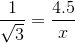Solving for, we get: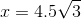Now, since, we know thatmust be smaller than. This means that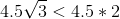or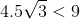Therefore, quantity B is larger than quantity A.

### All GRE Math Resources